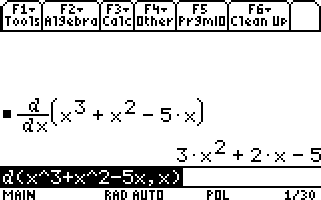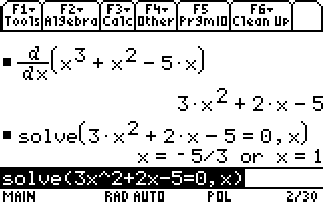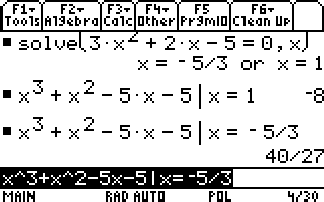# Knowledge Base

## Solution 11751: Finding Critical Points Using the TI-89 Family, TI-92 Family, and Voyage™ 200 Graphing Calculators.

### How can critical points be found using the TI-89 family, TI-92 family or Voyage 200?

The critical points of a function are the points where the derivative is equal to zero, reach a local minimum, or reach a local maximum. The following illustrates how to use the differentiate and solve functions to find critical points.

Example: Find the critical points using the solver and differentiation commands on the home screen for the following function:

f(x)= x^3+x^2-5x-5

The first step to finding the critical points is to differentiate the above function:

1) Press [F3] and select d( and enter d(x^3+x^2-5x-5,x)

2) Press [ENTER] to differentiate the following function. The calculator will return: 3x^2+2x-5To find one of the critical points you will need to set the derivative (shown above) equal to zero and solve for x:

1) Press [F2] and select solve( and enter solve(3x^2+2x-5=0,x)

This will solve for the unknown value, x, and return the following :x=1 or x= -5/3.Since there are two answers for the following function, there will be two sets of critical points on this function.

To solve for the additional points, plug x into the original equation:

1) From the home screen, enter what is shown below and press [ENTER].

x^3+x^2-5x-5| x=1

The displayed answer will show -8.

x^3+x^2-5x-5|x=-5/3

The displayed answer will show 40/27.The answers show the y coordinates for the two critical points on this function.
The critical values for the function f(x)= x^3+x^2-5x-5 are (-5/3,40/27) and (1,-8).

Please see the TI-89 family, TI-92 family and Voyage 200 guidebooks for additional information.